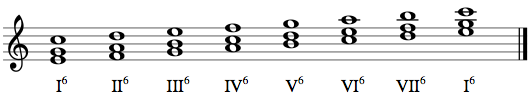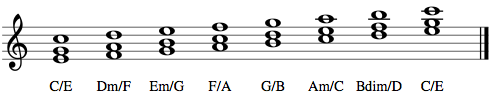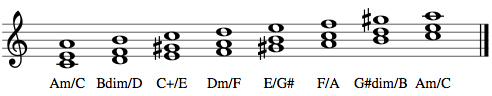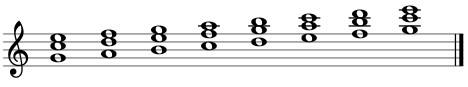## Topic outline

•### Welcome!

With this course you can practise:

Recommended to study before this course:

• Chords 3
•### 1st Inversion (chord degree)

A chord is in root position if its root is the lowest note. For example, the root of a C-major triad is C, so a C-major triad will be in root position. In an inverted chord, the root is not the lowest note. The inversions are numbered in the order their lowest notes appear in a close root-position chord (from bottom to top). (Wikipedia)

C-major triad (or any chord with three notes) has root position and two inversions:• In the first inversion, the lowest note is E – the third of the triad – with the fifth and the root stacked above it (the root now shifted an octave higher), forming the intervals of a minor third and a minor sixth above the inverted bass of E, respectively.
• In the second inversion, the lowest note is G – the fifth of the triad – with the root and the third above it (both again shifted an octave higher), forming a fourth and a sixth above the (inverted) bass of G, respectively. (Wikipedia)

The following C-major triads are all in the first inversion (third as bass):1. inversions of all triads in C major (sixth chords):•### 1st Inversion (chord symbols)

A notation for chord inversion often used in popular music is to write the name of a chord followed by a forward slash and then the name of the bass note. This is called a slash chord. For example, a C-major chord in first inversion (i.e., with E in the bass) would be notated as C/E. (Wikipedia)

1. inversion triads in C major:1. inversion triads in A minor:•### 2nd Inversion

In the second inversion, the lowest note is the fifth of the triad with the root and the third above it, forming a fourth and a sixth above the (inverted) bass. (Wikipedia)

A notation for chord inversion often used in popular music is to write the name of a chord followed by a forward slash and then the name of the bass note. This is called a slash chord. For example, a C-major chord in first inversion (i.e., with E in the bass) would be notated as C/E. (Wikipedia)

2. inversions of triads in C major: